# Image frequencies

hollowman
In digital electronics -- and notably digital reconstruction filters, Shannon and Nyquist theorems -- one encounters "images" (or frequency images).

In digital audio, for example, after the DAC (digital-to-analog converter), one must filter the output of the DAC with an anti-imaging filter (e.g., a low-pass filter).

I've encountered the phenomenon of images in textbook discussions. I'm not clear as to how and why the arise in Nature? I don't think they are like harmonics (which diminish in power (amplitude) the further get from the main freq.)

Simply stated, if I created a single-tone audio pulse (impulse), how and why are its image(s) created?

From wikipedia:
Sampled data reconstruction filters

The sampling theorem describes why the input of an ADC requires a low-pass analog electronic filter, called the anti-aliasing filter: the sampled input signal must be bandlimited to prevent aliasing (here meaning waves of higher frequency being recorded as a lower frequency).

For the same reason, the output of a DAC requires a low-pass analog filter, called a reconstruction filter - because the output signal must be bandlimited, to prevent imaging (meaning Fourier coefficients being reconstructed as spurious high-frequency 'mirrors'). This is an implementation of the Whittaker–Shannon interpolation formula.

Implementation
While in theory a DAC outputs a series of discrete Dirac impulses, in practice, a real DAC outputs pulses with finite bandwidth and width. Both idealized Dirac pulses, zero-order held steps and other output pulses, if unfiltered, would contain spurious high-frequency content when compared to the original signal. Thus, the reconstruction filter smooths the waveform to remove image frequencies (copies) above the Nyquist limit. In doing so, it reconstructs the continuous time signal (whether originally sampled, or modeled by digital logic) corresponding to the digital time sequence.

From Ken Pohlmann's Principles of Digital Audio (5th), here are some figures and their captions. I've emboldened all occurrences of term "image":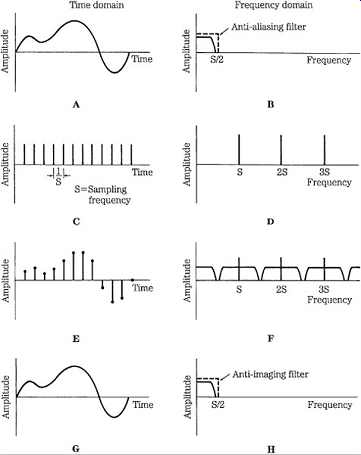FGR. 2 Time domain (left column) and frequency domain (right column) signals illustrate the process of bandlimited waveform sampling and reconstruction. A. Input signal after anti-aliasing filter. B. Spectrum of input signal. C. Sampling signal. D. Spectrum of the sampling signal. E. Sampled input signal. F. Spectrum of the sampled input signal. G. Output signal after anti-imaging filter. H. Spectrum of the output signal.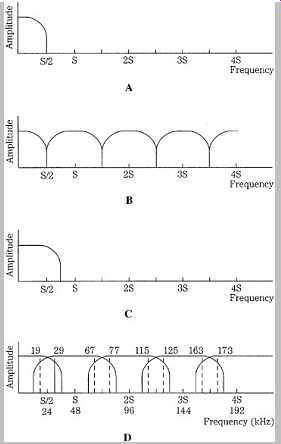FGR. 4 Spectral views of correct sampling and incorrect sampling causing aliasing. A. An input signal bandlimited to the Nyquist frequency. B. Upon reconstruction, images are contained within multiples of the Nyquist frequency. C. An input signal that is not bandlimited to the Nyquist frequency. D. Upon reconstruction, images are not contained within multiples of the Nyquist frequency; this spectral overlap is aliasing; For example, a 29-kHz signal will alias in a 48-kHz sampler.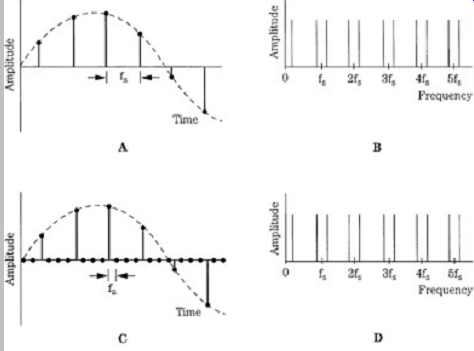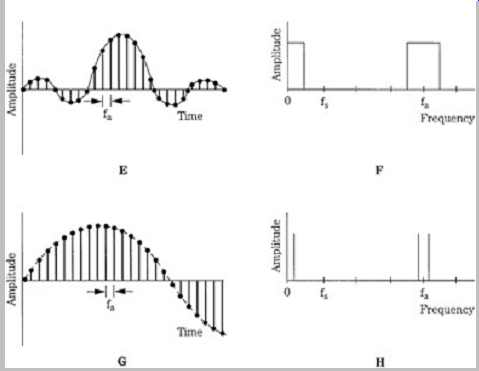FGR. 12 An oversampling filter resamples and interpolates the signal, using the impulse response; this is shown in the time domain (left column) and frequency domain (right column). A. The input signal is sampled at fs. B. The signal spectrum has images centered around multiples of fs. C. With resampling, zero-valued samples are placed between original samples at some interpolation ratio. D. The spectrum of the oversampled signal is the same as the original signal spectrum. E. The values of a sampled impulse response correspond to the coefficients of the digital filter. F. The transfer function of the filter shows passbands in the audio band and oversampling band. G. The digital filter performs interpolation to form new output sample values. H. The output-filtered signal has images centered around multiples of the oversampling frequency, fa.

#### Attachments

Homework Helper
I don't think they arise in nature very often.
In those cases when sampling is being done, there is some sort of low pass filter inherent in the system. For example, we don't see this type of artifact in eyesight because of a combination of factors that act as a low pass spatial filter - the saccadic eye movement; the size of the rods and cones; and the ability to focus precisely on the fovea compared to the distance between cones in that area.
In the case of human hearing, the sampling is done in the frequency domain - thus bypassing the issue completely.

•dlgoff
Gold Member
I don't think they arise in nature very often.
In those cases when sampling is being done, there is some sort of low pass filter inherent in the system. For example, we don't see this type of artifact in eyesight because of a combination of factors that act as a low pass spatial filter - the saccadic eye movement; the size of the rods and cones; and the ability to focus precisely on the fovea compared to the distance between cones in that area.
In the case of human hearing, the sampling is done in the frequency domain - thus bypassing the issue completely.
The Moire Effect when looking through a fine net curtain is one example, also seen on analogue TV screens where the scan lines effectively sample the vertical information. The process of sampling is akin to the electronic idea of mixing (using a diode etc) and beats. In such a case we see a double sideband version of the information based around each oscillator harmonic.

hollowman
Below is an excerpt from another textbook on Digital Audio (The Art of Digital Audio ):
======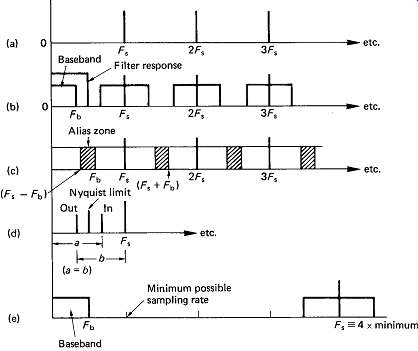FGR. 4 (a) Spectrum of sampling pulses. (b) Spectrum of samples. (c) Aliasing due to sideband overlap. (d) Beat-frequency production. (e) 4x oversampling.

In the same way that AM radio produces sidebands or images above and below the carrier, sampling also produces sidebands although the carrier is now a pulse train and has an infinite series of harmonics as shown in FGR. 4(a). The sidebands repeat above and below each harmonic of the sampling rate as shown in (b).
=======
In FIGR. 4a, note how the "infinite series of harmonics " (i.e., images) maintain constant amplitude? Shouldn't the image amplitudes fade out naturally as the freq. gets further from Fs (i.e., original sampling freq.)? Or am I not understanding something fundamental?

#### Attachments

hollowman
Just adding some more topical content...https://www.maximintegrated.com/en/app-notes/index.mvp/id/928#
"Note the data is replicated at multiples of the sample rate (these are 'images' of the original signal); this is a fundamental characteristic of sampled data systems. "

Also:

https://www.maximintegrated.com/en/app-notes/index.mvp/id/3853Figure 2. The ideal output from a DAC is a train of voltage impulses in the time domain (a), and a series of image spectra in the frequency domain (b). Actual DACs use a zero-order hold to hold the output voltage for one update period (c), which causes output-signal attenuation by the sinc envelope (d).Figure 3. This representation of a DAC output in the frequency domain shows that the desired signal is generally within the first Nyquist zone, but many image signals are present at higher frequencies.

Gold Member
The sampling harmonics decrease with frequency, like those from any repetitive pulse. If the sampling pulse were rectangular, only odd harmonics would be present. In practice all harmonics are likely to be present.

Gold Member
Other definitions for 'image' are 'frequency folding' and 'aliasing'. Here are a couple of images that show what happens. The vertical pink lines are the sampling points. They are at the same rate in both images. The first image is sampled at twice the signal frequency, the Nyquist rate.

In this first image the signal is sampled at both the positive and negative peaks. The sampled values are +4, -4, +4, -4, +4, -4.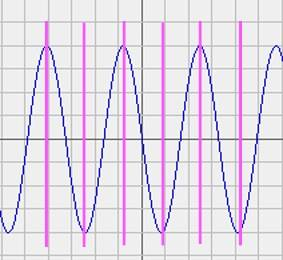This second signal is three times the frequency of the first one above.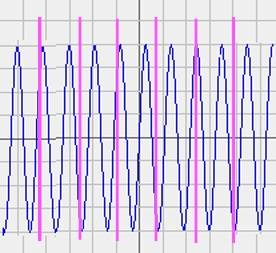The signal is again sampled at the positive and negative peaks. The sampled values are +4, -4, +4, -4, +4, -4, the same as above. But there is an extra cycle between the sample points that doesn't show up in the data![/b]

So, if you don't want extra high-frequency noise in the digitized data, filter it out beforehand. You can't do it afterwards.

#### Attachments

hollowman
So, if you don't want extra high-frequency noise in the digitized data, filter it out beforehand. You can't do it afterwards.
I assume you mean in during the A/D process: i.e., use an anti-aliasing filter before the ADC.
Actually, modern ADC systems (e.g., as used for digital audio) use an analog anti-aliasing filter before the A/D converter (an IC chip). Then, following the A/D converter, an oversampling digital filter is used to "filter out" garbage introduced in the A/D.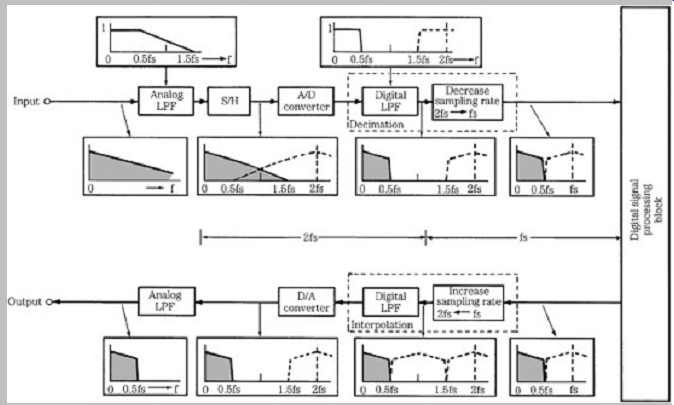#### Attachments

•Tom.G